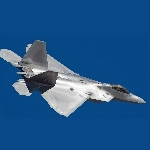Community Profile# ChristianW

Active since 2013

M.Sc. Aeronautics and Astronautics Student in flow with MATLAB.
- 360° learning -

#### Statistics

All
••••#### Content Feed

View by

assigning a a structured 2x2 matrix using a loop
M = 5; centroid = rand(M,2); detresp = struct('a',num2cell(centroid,2),'c','d'); or detresp = struct('a',cell...

ongeveer 9 jaar ago | 0

The function "nchoosek" is not working in my code
cnt{1}=[3;1;3;2;1;2] cnt{2}=[2;3;1;3;1;2] value = cellfun(@(x) nchoosek(x(x~=1),2),cnt,'un',0); or with loop ...

ongeveer 9 jaar ago | 0

How can I have editable text inside a listbox?
<http://www.mathworks.de/matlabcentral/fileexchange/24861-41-complete-gui-examples Matt Fig GUI Examples> Search for listbox ...

ongeveer 9 jaar ago | 0

Sharing Three Dimensional Array Variable Among GUIs
In a GUIDE its provided to use the handles structure. But, using global does also work. You need to declare the variable as g...

ongeveer 9 jaar ago | 0

Roulette Algorithm Probability Loop
To summarize the strategie: *You allways lose all your money.* This is for 37 slots, only one zero slot. bet0 = 5; % ...

ongeveer 9 jaar ago | 0

Reading many files in one loop
doc dir D = dir([myfolder 'my*file*name*.*']); files = {D.name}';

ongeveer 9 jaar ago | 0

How to make a signal vector?
S = [S1;S2]

ongeveer 9 jaar ago | 0

| accepted

How to stop a for loop execution from GUI?(From Toolbar stop button)
You can write your flag in the |UserData|-property of the toolbaricon. ... set(handles.uitoolbar1,'UserData',0) for ....

ongeveer 9 jaar ago | 0

| accepted

Calculate average of multiple matrices by reading an m.file
k = 0; while (reading file) ... k = k+1; match(k,:) = strcmp('Hello', out) end M = mean(match)

ongeveer 9 jaar ago | 0

| accepted

function test_main % Plots graph and sets up a custom data tip update function fig = figure('DeleteFcn','doc datacursorm...

ongeveer 9 jaar ago | 14

| accepted

Importing text from Excel sheets
Here's an example on how to use fprintf with cells: C{1} = sprintf('Hello\n'); c = {'A','B','C';1,2,3}; C{2} = sprint...

ongeveer 9 jaar ago | 0

| accepted

Wrong way to index: Index exceeds matrix dimensions
max(Z) is greater then 2270?

ongeveer 9 jaar ago | 0

| accepted

How do i plot a bounding box around the output of a Moving object algorithm?
With Image Processing Toolbox: doc regionprops An example: BW = imread('text.png'); imshow(BW) bb = regionprops...

ongeveer 9 jaar ago | 0

Plot legend with multiple fitresult curves
x = 1:10; y = sin(x); f = fit(x',y','cos(x+a)'); h1 = plot(x,y,'ok'); hold on h2 = plot(f); X = 1:0.1:20; %...

ongeveer 9 jaar ago | 0

The trapz inputs are just points. But quad needs a function as input. x = 0:1:10; y = sin(x); A_trapz = trapz(x,y) ...

ongeveer 9 jaar ago | 0

open wav file error
myFile=[PathName,FileName];

ongeveer 9 jaar ago | 0

| accepted

doc isinteger isinteger(int8(3))

ongeveer 9 jaar ago | 0

How can I create draggable lines in a BW-Mask in an image overlay?
This might be usefull for you: function test close all imagesc(peaks), axis off, axis image, hold on d = [10 25 20...

ongeveer 9 jaar ago | 0

| accepted

How to use image sc and contour?
n = 20; % 2000 takes long calculation time fot contour X = randi(1000,n); [C,h] = contour(X,[400 600]); axis image se...

ongeveer 9 jaar ago | 0

Read image and save it using imwrite?
Input_folder = '.\'; % folder with big images Output_folder = 'c:\result'; D = dir([Input_folder 'image*_big.bmp']); ...

ongeveer 9 jaar ago | 2

| accepted

for loop with stored variables
There are several ways. I would make for the Plantary Temperature (Te) a new function, and vectorize Albedo (A). Like this: ...

ongeveer 9 jaar ago | 0

| accepted

From logical to binary ?
Try w=char(bin2dec(reshape(num2str(w),8,[]).')).';

ongeveer 9 jaar ago | 0

| accepted

Nested for loop in parfor, indexing
You could use a seperated variable for the inner loop. On the example of x: X = zeros(M,N); %preallocate parfor i=1:M ...

ongeveer 9 jaar ago | 0

| accepted

Help in Syntax of Eval and Sprintf
Y = cell(size(mydate1)); % preallocate for k = 1:length(mydate1) str = [mydate1{k} '::' mydate2{k}]; % Date String R...

ongeveer 9 jaar ago | 0

| accepted

Apollo Capsule 3D Model
doc patch Depending on your model complexity, you could use 3D CAD software and save as *.stl. There's a function called rn...

ongeveer 9 jaar ago | 0

Elementwise diff() with an condition
X = {[1,2,4,5],[23,12,13]}; Y = cellfun(@diff,X,'un',0); B = 1.5; Ycut = cellfun(@(x) x(abs(x)>B),Y,'un',0);

ongeveer 9 jaar ago | 1

| accepted

How to use an Xbox Controller in Matlab for real time signal processing?
With Simulink 3D Animation there is a <http://www.mathworks.de/de/help/sl3d/joystickinput.html Joystick Block>.

ongeveer 9 jaar ago | 1

| accepted

I tried to solve this system of ODE and it took so long to give me results.
Pretty sure your function hang up in the while loops. I guess it should be IF instead of WHILE. while 2<r This is an en...

ongeveer 9 jaar ago | 0

| accepted